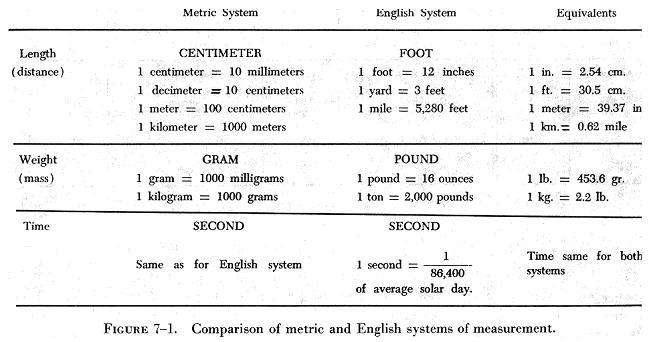MATTER MATTER

Although matter is the most basic of all things related to the field of physics and the material world, it is the hardest to define. Since it cannot be rigidly defined, this chapter will point out those characteristics which are easily recognizable.

Matter itself cannot be destroyed, but it can be changed from one state into another state by chemical or physical means. It is usually considered in terms of the energy it contains, absorbs, or gives off. Under certain controlled conditions, it can be made to aid man in his everyday life.

Matter is any substance that occupies space and has weight. There are three states of matter: (1) Solids, (2) liquids, and (3) gases. Solids have a definite volume and a definite shape; liquids have a definite volume, but they take the shape of the containing vessel; gases have neither a definite volume nor a definite shape. Gases not only take the shape of the containing vessel, but they expand and fill the vessel, no matter what its volume.

Water is a good example of matter changing from one state to another. At high temperature it is in the gaseous state known as steam. At moderate temperatures it is a liquid, and at low temperatures it becomes ice, a solid state. In this example, the temperature is the dominant factor in determining the state that the substance assumes. Pressure is another important factor that will effect changes in the state of matter. At pressures lower than atmospheric, water will boil and thus change into steam at temperatures lower than 212° F. Pressure is a critical factor in changing some gases to liquids or solids. Normally, when pressure and chilling are both applied to a gas, it assumes a liquid state. Liquid air, which is a mixture of oxygen and nitrogen, is produced in this manner.

Characteristics of Matter

All matter has certain characteristics or general properties. These properties are defined elementally and broadly at this point, and more specifically in applications throughout the text. Among these properties and relationships are:

a. Volume - meaning to occupy space; having some measurements such as length, width, and height. It may be measured in cubic inches, cubic centimeters, or the like.

b. Mass - the measurement of quantity or the measure of the quantity of matter in a body. Mass does not vary even though the state changes.

c. Attraction - a force acting mutually between particles of matter, tending to draw them together. Sir Isaac Newton called this the "Law of Universal Gravitation." He showed how each particle of matter attracts every other particle, how people are bound to the earth, and how the planets are attracted in the solar system.

d. Weight - the measure of universal gravitation. The pull of gravity on a body is called the weight of the body and indicates how heavy the body is.

e. Density - the mass (weight) of a substance per unit volume. Density can be used to distinguish various types of matter. If a substance is very dense, a large quantity of this matter would occupy a small volume.

f. Inertia - the opposition which a body offers to any change of motion. The property of inertia is common to all matter. It is best expressed in Newton's first law: "A body at rest remains at rest, and a body in motion continues to move at constant speed along a straight line, unless the body is acted upon in either case by an external force."

g. Porosity - having pores or spaces where smaller particles may fit when a mixture takes place. This is sometimes referred to as granular; consisting or appearing to consist of small grains or granules.

h. Impenetrability - simply stated means that no two objects can occupy the same place at the same time. Thus, two portions of matter cannot at the same time occupy the same space.

Matter may be classified as either an element or a compound, depending upon the complexity of its structure. An element is matter that cannot be reduced chemically into a simpler substance. A compound is matter formed by some combination of elements.

Two basic particles, the atom and the molecule, make up all matter. The molecule is the smallest particle of a substance which still has all the properties of the original substance. In physics the molecule is the unit of matter. The atom is the smallest particle of an element that can combine with other atoms to form molecules. In chemistry, the atom is the unit of matter.

While the subject of matter may seem complex, it is difficult to think of anything simpler than matter. It can be referred to as "anything that occupies space."

Systems of Measurement

The two most commonly used systems of measurement are the English system, which is still in general use in the United States, and the metric system, used in most European countries and now adopted by the Armed Forces of the United States. The metric system is normally used in all scientific applications.

The three basic quantities which require units of measurement are mass (weight), length (distance), and time.

The metric system is sometimes called the CGS system because it uses as basic measuring units, the centimeter (C) to measure length, the gram (G) to measure mass, and the second (S) to measure time.

The English system uses different units for the measurement of mass and length. The pound is the unit of weight; the foot is used to measure length. The second is used to measure time as in the metric system.

The units of one system can be converted to units in the other system by using a conversion factor or by referring to a chart similar to that shown in figure 7-1. In this figure the English and the metric systems are compared; in addition, a column of equivalents is included which can be used to convert units from one system to the other.